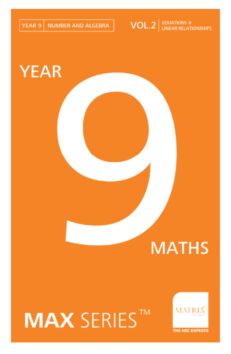Year 9 Maths Max Series Vol 2: Equations & Linear Relationships Revision Workbook

(1 customer review)

\$32.00

In stock

SKU: XM09V2-1 Categories: ,

Ace your Year 9 Equations and Linear Relationships exams with this 128-page exam workbook!

Do you worry about your child’s Maths exam skills? Many students are caught off-guard by questions that appear in exams that they haven’t seen before. Does your child struggle to use classroom skills in their exams? They’re not alone. Students often struggle to translate what they learn from class and school textbooks to their exams.

Conventional school textbooks teach course content to give students a sound knowledge and understanding of key fundamental concepts. But for exams, this isn’t enough.

Why is this not enough to achieve exam success at school? At school, students are required to:

• Demonstrate extensive knowledge and skills
• Apply Maths concepts to unfamiliar questions/situations
• Use sophisticated multi-step reasoning
• Exhibit excellent problem-solving skills

Here’s how we get students exam ready!

Matrix has 20 years of experience preparing thousands of students from Year 7 through Year 12 to ace their exams and HSC. We’ve drawn on this experience and track record of successful teaching to create the Year 9 Maths Max SeriesTM  Volume 2. Help your child prepare for their next exam with the knowledge and processes we’ve developed.

The Year 9 Maths Max SeriesTM  Volume 2 is not your typical Year 9 Maths Revision Workbook. This workbook is designed for exam preparation, as well as providing students with the opportunity to further develop and practise fundamental concepts.

When students use this Maths revision workbook correctly, they will gain significant advantages in their school exams.

The Year 9 Maths Max SeriesTM  Volume 2 empowers students by:

• Giving them the confidence to take control of their learning
• Exposing students to exam-style questions to reduce the chance of encountering unfamiliar problems
• Effectively preparing students for school exams by bridging the knowledge and skills gap between their textbooks and exam questions
• Improving their skills by sharing the exam and problem-solving techniques not published in regular textbooks.How to use the Year 9 Maths Max SeriesTM Volume 2 for Maths exam success

Exam success comes from doing, not just knowing. This book is designed for students who have a sound understanding of the key year 9 concepts of:

• Equations
• Linear relationships

You need more than a sound understanding of these concepts. Students need effective practice to succeed in exams. The Year 9 Maths Max SeriesTM  Volume 2 will get you exam ready.

Students should only use this Year 9 Maths revision workbook after gaining a sound knowledge and understanding of the key concepts of the Year 9 Mathematics course content for Equations and Linear Relationships. This Maths revision workbook will effectively prepare students for school exams when the following steps are closely followed:

Step 1: Review theory content

At the beginning of each topic, there is a concise summary of the key concepts. Be sure to read and understand it before attempting any questions.Step 2: Study the examples and their solutions to gain clarity

The examples expose students to various exam-style questions. The step-by-step worked solutions for each example provides clear multi-step reasoning to enhance your understanding and provide a structure for maximising marks in exams.

Step 3: Attempt practice questions to improve problem-solving skills

Questions are organised in a progressive manner to facilitate independent learning.

If you have difficulty, the ‘NOTES TO STUDENTS’ section provides you with hints to encourage problem-solving without referring to the solution.

The ‘LEVEL’ of difficulty is a useful tool to assess your ability to solve new, unseen and difficult problems.This Year 9 Maths revision workbook is compliant with the NESA NSW Mathematics Stage 5.2 and 5.3 Syllabus.

The Maths revision content in the Year 9 Maths Max SeriesTM Volume 2 covers the topics ‘Equations and Linear Relationships’ from the ‘Number & Algebra’ strand of the NSW Mathematics Stage 5.2 and 5.3 Syllabus.

 Strand Topics Content Number and Algebra Equations Solving linear equations Rearranging formulas Rearranging equations Using equations to solve word problems Solving and graphing linear inequalities Linear Relationships Finding the midpoint and gradient of intervals Finding the distance between two points Graphing linear equations Finding the equation of a line parallel or perpendicular to a given line Solving simultaneous equations Using simultaneous equations to solve word problems

For detailed information including the contents of the topics, please visit the NESA website.

1 review for Year 9 Maths Max Series Vol 2: Equations & Linear Relationships Revision Workbook

1.Michael

awesome!You're viewing: Year 9 Maths Max Series Vol 2: Equations & Linear Relationships Revision Workbook \$32.00